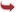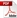International Journal of Scientific and Research Publications

#### IJSRP, Volume 2, Issue 8, August 2012 Edition [ISSN 2250-3153]Fuzzy Shortest Route Algorithm for Telephone Line Connection
Dr. G.Nirmala, K.Uma
Abstract: In computer science , there are many algorithm that finds a minimum spanning tree for a connected weighted undirected fuzzy graph. This means it finds a subset of the edge-set that forms a fuzzy spanning tree that includes every vertex, where the total weight of all the edges in the fuzzy tree is minimized. Generally algorithm has many applications, such as landline (intercom) phone connection to a particular college in order to minimize the shortest distance, which applies Nearest Neighbor Algorithm, Sorted Edges Algorithm, Kruskal’s Algorithm to a randomly weighted fuzzy graph. In this paper, we find the solution for the problem, that A.V.V.M. Sri Pushpam College, (Autonomous), Poondi, Thanjavur District, South India need to connect updated intercom lines connecting all the departments using fuzzy shortest route algorithm. The problem is to minimize the amount of new line using Kruskal’s Algorithm with fuzzy graph. These algorithm continuously increases the size of a fuzzy tree, one edge at a time, starting with a fuzzy tree consisting of a single vertex, until it spans all vertices of the fuzzy graph.
[VIEW FULL PAPER][DOWNLOAD]

Reference this Research Paper (copy & paste below code):

Dr. G.Nirmala, K.Uma (2018); Fuzzy Shortest Route Algorithm for Telephone Line Connection; Int J Sci Res Publ 2(8) (ISSN: 2250-3153). http://www.ijsrp.org/research-paper-0812.php?rp=P0790